# Important Questions CBSE Class 9 Maths Chapter 7- Triangles

Important Questions for CBSE Class 9 Chapter 7 -Triangles are provided here by our experts, along with their solutions. These questions are extracted from the NCERT book as per CBSE syllabus. Students who are preparing for standard 9 Maths final exam (2022 – 2023) should practise these questions to score excellent marks.

Solve extra questions along with important questions for class 9 Maths to have a better practice and brief revision before the examination. The questions in Chapter 7, triangles, are majorly based on congruency or similarity of triangles. Solving these questions will give students an idea of the types of questions asked in the exam.

Also Check:

## Important Questions & Solutions For CBSE Class 9 Chapter 7 (Triangles)

Q.1: ABCD is a quadrilateral in which AD = BC and ∠DAB = ∠CBA. Prove that

(i) ΔABD ≅ ΔBAC

(ii) BD = AC

(iii) ∠ABD = ∠BAC.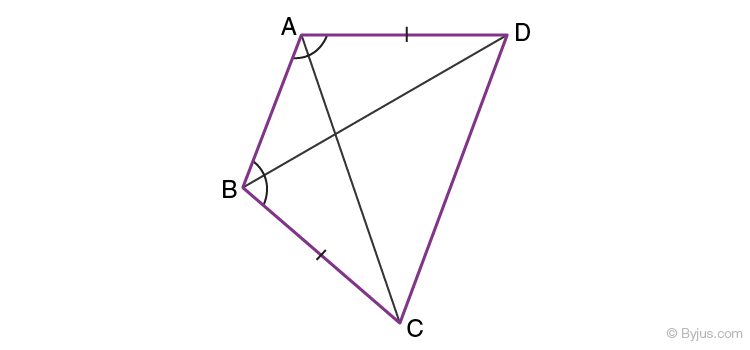Solution:

As per given in the question,

∠DAB = ∠CBA and AD = BC.

(i) ΔABD and ΔBAC are similar by SAS congruency as

AB = BA (common arm)

∠DAB = ∠CBA and AD = BC (given)

So, triangles ABD and BAC are similar

i.e. ΔABD ≅ ΔBAC. (Hence proved).

(ii) As it is already proved,

ΔABD ≅ ΔBAC

So,

BD = AC (by CPCT)

(iii) Since ΔABD ≅ ΔBAC

So, the angles,

∠ABD = ∠BAC (by CPCT).

Q.2: AD and BC are equal perpendiculars to a line segment AB. Show that CD bisects AB.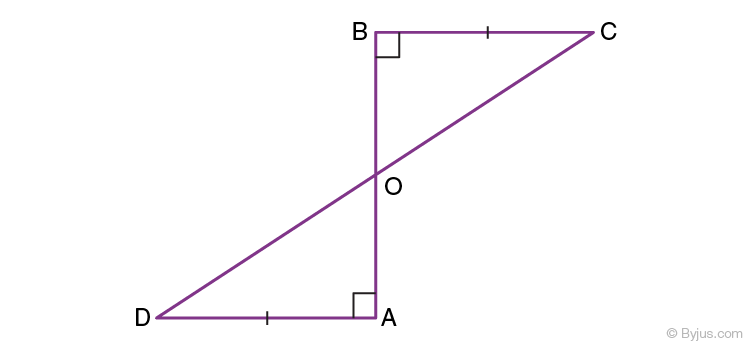Solution:

Given, AD and BC are two equal perpendiculars to AB.

To prove: CD is the bisector of AB

Proof:

Triangles ΔAOD and ΔBOC are similar by AAS congruency

Since:

(i) ∠A = ∠B (perpendicular angles)

(iii) ∠AOD = ∠BOC (vertically opposite angles)

∴ ΔAOD ≅ ΔBOC.

So, AO = OB ( by CPCT).

Thus, CD bisects AB (Hence proved).

Q.3: Line l is the bisector of an angle ∠A and B is any point on l. BP and BQ are perpendiculars from B to the arms of ∠A. Show that:

(i) ΔAPB ≅ ΔAQB

(ii) BP = BQ or B is equidistant from the arms of ∠A.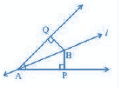Solution:

It is given that the line “l” is the bisector of angle ∠A and the line segments BP and BQ are perpendiculars drawn from l.

(i) ΔAPB and ΔAQB are similar by AAS congruency because;

∠P = ∠Q (both are right angles)

AB = AB (common arm)

∠BAP = ∠BAQ (As line l is the bisector of angle A)

So, ΔAPB ≅ ΔAQB.

(ii) By the rule of CPCT, BP = BQ. So, we can say point B is equidistant from the arms of ∠A.

Q.4: AB is a line segment and P is its mid-point. D and E are points on the same side of AB such that ∠BAD = ∠ABE and ∠EPA = ∠DPB. Show that

(i) ΔDAP ≅ ΔEBP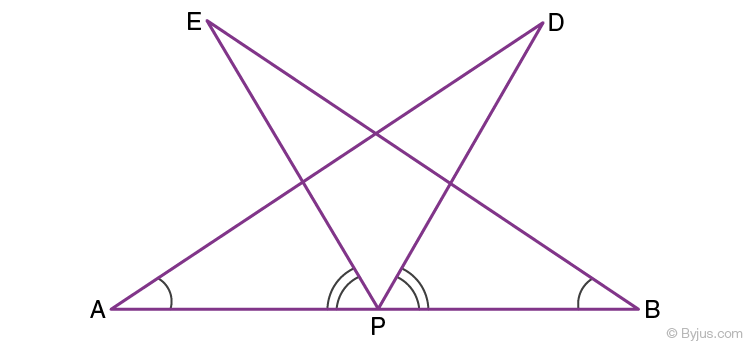Solution:

Given, P is the mid-point of line segment AB.

Also, ∠BAD = ∠ABE and ∠EPA = ∠DPB

(i) Given, ∠EPA = ∠DPB

Now, add ∠DPE on both sides,

∠EPA + ∠DPE = ∠DPB + ∠DPE

This implies that angles DPA and EPB are equal

i.e. ∠DPA = ∠EPB

Now, consider the triangles DAP and EBP.

∠DPA = ∠EPB

AP = BP (Since P is the mid-point of the line segment AB)

So, by ASA congruency criterion,

ΔDAP ≅ ΔEBP.

(ii) By the rule of CPCT,

Q.5: In right triangle ABC, right-angled at C, M is the mid-point of hypotenuse AB. C is joined to M and produced to a point D such that DM = CM. Point D is joined to point B (see the figure). Show that:

(i) ΔAMC ≅ ΔBMD

(ii) ∠DBC is a right angle.

(iii) ΔDBC ≅ ΔACB

(iv) CM = 1/2 AB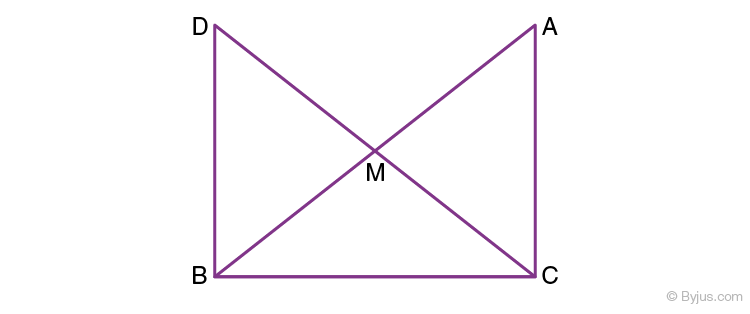Solution:

It is given that M is the mid-point of the line segment AB, ∠C = 90°, and DM = CM

(i) Consider the triangles ΔAMC and ΔBMD:

AM = BM (Since M is the mid-point)

CM = DM (Given)

∠CMA = ∠DMB (Vertically opposite angles)

So, by SAS congruency criterion, ΔAMC ≅ ΔBMD.

(ii) ∠ACM = ∠BDM (by CPCT)

∴ AC ∥ BD as alternate interior angles are equal.

Now, ∠ACB + ∠DBC = 180° (Since they are co-interiors angles)

⇒ 90° + ∠B = 180°

∴ ∠DBC = 90°

(iii) In ΔDBC and ΔACB,

BC = CB (Common side)

∠ACB = ∠DBC (Both are right angles)

DB = AC (by CPCT)

So, ΔDBC ≅ ΔACB by SAS congruency.

(iv) DC = AB (Since ΔDBC ≅ ΔACB)

⇒ DM = CM = AM = BM (Since M the is mid-point)

So, DM + CM = BM + AM

Hence, CM + CM = AB

⇒ CM = (½) AB

Q.6: ABC is an isosceles triangle in which altitudes BE and CF are drawn to equal sides AC and AB respectively. Show that these altitudes are equal.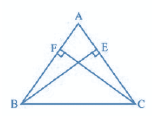Solution:

Given:

(i) BE and CF are altitudes.

(ii) AC = AB

To prove:

BE = CF

Proof:

Triangles ΔAEB and ΔAFC are similar by AAS congruency, since;

∠A = ∠A (common arm)

∠AEB = ∠AFC (both are right angles)

AB = AC (Given)

∴ ΔAEB ≅ ΔAFC

and BE = CF (by CPCT).

Q.7: ΔABC is an isosceles triangle in which AB = AC. Side BA is produced to D such that AD = AB. Show that ∠BCD is a right angle.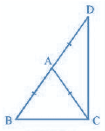Solution:

Given, AB = AC and AD = AB

To prove: ∠BCD is a right angle.

Proof:

Consider ΔABC,

AB = AC (Given)

Also, ∠ACB = ∠ABC (Angles opposite to equal sides)

Now, consider ΔACD,

Also, ∠ADC = ∠ACD (Angles opposite to equal sides)

Now,

In ΔABC,

∠CAB + ∠ACB + ∠ABC = 180°

So, ∠CAB + 2∠ACB = 180°

⇒ ∠CAB = 180° – 2∠ACB — (i)

∠CAD = 180° – 2∠ACD — (ii)

Also,

∠CAB + ∠CAD = 180° (BD is a straight line.)

Adding (i) and (ii) we get,

∠CAB + ∠CAD = 180° – 2∠ACB + 180° – 2∠ACD

⇒ 180° = 360° – 2∠ACB – 2∠ACD

⇒ 2(∠ACB + ∠ACD) = 180°

⇒ ∠BCD = 90°

Q.8: ΔABC and ΔDBC are two isosceles triangles on the same base BC and vertices A and D are on the same side of BC (see the figure). If AD is extended to intersect BC at P, show that

(i) ΔABD ≅ ΔACD

(ii) ΔABP ≅ ΔACP

(iii) AP bisects ∠A as well as ∠D.

(iv) AP is the perpendicular bisector of BC.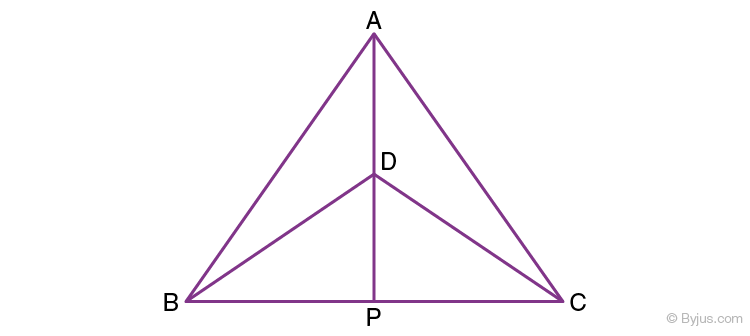Solution:

In the above question, it is given that ΔABC and ΔDBC are two isosceles triangles.

(i) ΔABD and ΔACD are similar by SSS congruency because:

AB = AC (Since ΔABC is isosceles)

BD = CD (Since ΔDBC is isosceles)

∴ ΔABD ≅ ΔACD.

(ii) ΔABP and ΔACP are similar as:

AP = AP (common side)

∠PAB = ∠PAC ( by CPCT since ΔABD ≅ ΔACD)

AB = AC (Since ΔABC is isosceles)

So, ΔABP ≅ ΔACP by SAS congruency.

(iii) ∠PAB = ∠PAC by CPCT as ΔABD ≅ ΔACD.

AP bisects ∠A. ………… (1)

Also, ΔBPD and ΔCPD are similar by SSS congruency as

PD = PD (It is the common side)

BD = CD (Since ΔDBC is isosceles.)

BP = CP (by CPCT as ΔABP ≅ ΔACP)

So, ΔBPD ≅ ΔCPD.

Thus, ∠BDP = ∠CDP by CPCT. ……………. (2)

Now by comparing equation (1) and (2) it can be said that AP bisects ∠A as well as ∠D.

(iv) ∠BPD = ∠CPD (by CPCT as ΔBPD ≅ ΔCPD)

and BP = CP — (1)

also,

∠BPD + ∠CPD = 180° (Since BC is a straight line.)

⇒ 2∠BPD = 180°

⇒ ∠BPD = 90° —(2)

Now, from equations (1) and (2), it can be said that

AP is the perpendicular bisector of BC.

Q.9: Two sides AB and BC and median AM of one triangle ABC are respectively equal to sides PQ and QR and median PN of ΔPQR (see the figure). Show that:

(i) ΔABM ≅ ΔPQN

(ii) ΔABC ≅ ΔPQR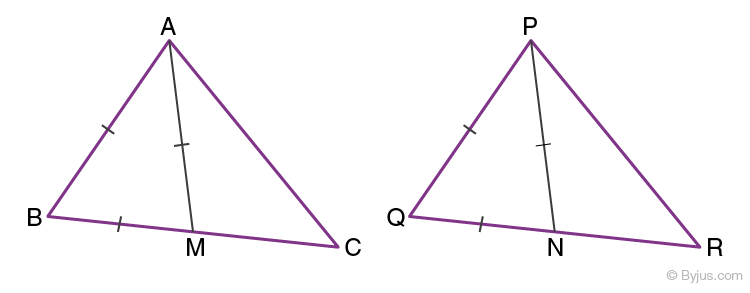Solution:

Given;

AB = PQ,

BC = QR and

AM = PN

(i) 1/2 BC = BM and 1/2QR = QN (Since AM and PN are medians)

Also, BC = QR

So, 1/2 BC = 1/2QR

⇒ BM = QN

In ΔABM and ΔPQN,

AM = PN and AB = PQ (Given)

∴ ΔABM ≅ ΔPQN by SSS congruency.

(ii) In ΔABC and ΔPQR,

AB = PQ and BC = QR (Given)

∠ABC = ∠PQR (by CPCT)

So, ΔABC ≅ ΔPQR by SAS congruency.

Q.10: In the Figure, PR > PQ and PS bisect ∠QPR. Prove that ∠PSR > ∠PSQ.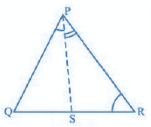Solution:

Given, PR > PQ and PS bisects ∠QPR

To prove: ∠PSR > ∠PSQ

Proof:

∠QPS = ∠RPS — (1) (PS bisects ∠QPR)

∠PQR > ∠PRQ — (2) (Since PR > PQ as angle opposite to the larger side is always larger)

∠PSR = ∠PQR + ∠QPS — (3) (Since the exterior angle of a triangle equals the sum of opposite interior angles)

∠PSQ = ∠PRQ + ∠RPS — (4) (As the exterior angle of a triangle equals to the sum of opposite interior angles)

∠PQR + ∠QPS > ∠PRQ + ∠RPS

Now, from (1), (2), (3) and (4), we get

∠PSR > ∠PSQ.

## Video Lesson on Congruent Triangles### Extra Questions for Class 9 Maths Triangles Chapter 7

1. Two adjacent angles on a straight line are in a ratio 5:4. Find the measure of each one of these angles. (Answer: 100 ° and 80 °degree).
2. Prove that bisectors of two adjacent supplementary angle include a right angle.
3. If two straight lines are perpendicular to the same line, prove that they are parallel to each other.
4. The angles of the triangle are in the ratio 2:3:7. Find the measure of each angle of the triangle. (Answer: 30°, 45° and 105°)
5. In triangle ABC , ∠A – ∠B = 33 degree and ∠B – ∠C = 180 degree.Find the measure of each angle of the triangle. (Answer: ∠A = 88° and ∠B = 55° and ∠C = 37°).
6. Of the three angles of the triangle, one is twice the smallest and another one is thrice the smallest. Find the angles. (Answer: 60°, 90°, 30°)
7. If each of a triangle is less than the sum of the other two, show that the triangle is acute-angled.
8. The sum of two angles of a triangle is 116° and their difference is 24. Find the measure of each angle of the triangle. (Answer: 46°, 70 °, 64°).
9. Two straight lines AB and CD cut each other at O. if angle BOD = 63° then find angle BOC. (Answer: 117°).
10. Two complementary angles are such that twice the measure of the one is equal to three times the measure of the other. The larger of the two measures is. (Answer: 54°).
11. Show that of all line segments drawn from a given point, not on it, the perpendicular line segment is the shortest.
12.  If the diagonals of the rectangle ABCD intersect at O and  ∠COD = 78°, then ∠OAB is:

a) 35o

b) 51o

c) 70o

d) 110o

13.  The measure of an angle is twice the measure of supplementary angle. What is the measure of angles?